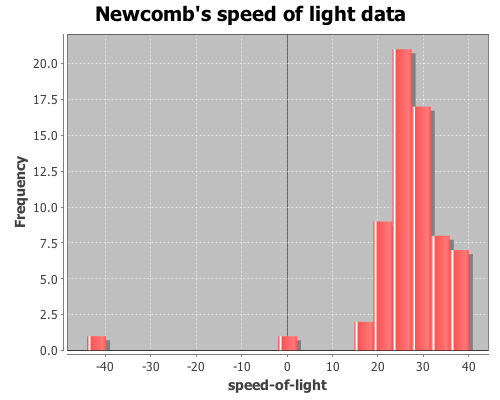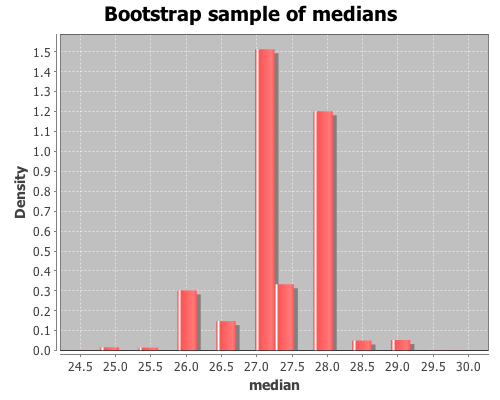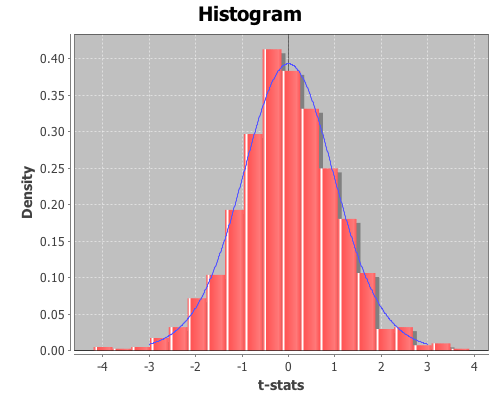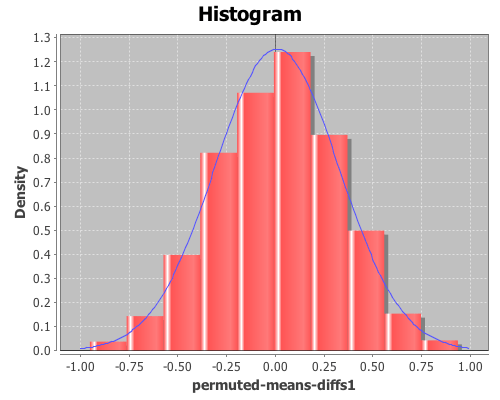# Category Archives: resampling

## Bootstrapping: estimating confidence intervals, standard errors, and bias

In addition to discovering Benford’s law (which I used in a previous post) 57 years before Frank Benford, Simon Newcomb made a series of measurements of the speed of light. In 1882 he measured the time in seconds that a light signal took to travel the 7,400 meters from the US Naval observatory, on the Potomac River, to a mirror at the base of the Washington Monument and back. Newcomb’s data are frequently used to demonstrate statistical techniques, as I will do here. The data are coded as deviations from 24,800 nanoseconds, so the first entry, corresponding to 24,828 nanoseconds, is 28.

Here’s a vector, x, containing the measurements.

``````(def x [28 -44 29 30 24 28 37 32 36
27 26 28 29 26 27 22 23 20
25 25 36 23 31 32 24 27 33
16 24 29 36 21 28 26 27 27
32 25 28 24 40 21 31 32 28
26 30 27 26 24 32 29 34 -2
25 19 36 29 30 22 28 33 39
25 16 23])``````

There are two obvious outliers in the data, so the median, not the mean, is the preferred estimate of location. Bootstrapping is a method often employed for estimating confidence intervals, standard errors, and estimator bias for medians.

``(use '(incanter core stats charts))``

View a histogram of the data, note the two outlier observations at -2 and -44.

``````(view (histogram x
:nbins 20
:title "Newcomb's speed of light data"))``````Now calculate the median of the sample data

``(median x)``

The result is 27, but how does this value relate to the speed of light? We can translate this value to a speed in meters/sec with the following function.

``````(defn to-speed
"Converts Newcomb's data into speed (meters/sec)"
([x] (div 7400 (div (plus 24800 x) 1E9))))
``````

Now apply it to the median.

``(to-speed (median x))``

The result is 2.981E8 m/sec (the true speed-of-light is now considered 2.9799E8 m/sec), but how good of an estimate of the median is this? How much sampling error is there? We can estimate confidence intervals, standard errors and estimator bias using bootstrapping.

Bootstrapping is a technique where items are drawn from a sample, with replacement, until we have a new sample that is the same size as the original. Since each item is replaced before the next item is drawn, a single value may appear more than once, and other items may never appear at all. The desired statistic, in this case median, is calculated on the new sample and saved. This process is repeated until you have the desired number of sample statistics. Incanter’s bootstrap function can be used to perform this procedure.

Draw 10,000 bootstrapped samples of the median.

``(def t* (bootstrap x median :size 10000))``

View a histogram of the bootstrap sample of the median

``````(view (histogram t*
:density true
:nbins 20
:title "Bootstrap sample of medians"
:x-label "median"))``````The bootstrap distribution is very jagged because the median is discrete, so there are only a small number of values that it can take. Later, I will demonstrate how to smooth over the discreteness of the median with the :smooth option to the bootstrap function.

The mean of t* is the bootstrap estimate of the median,

``(mean t*)``
``` ```

which is 27.301. An estimate of the 95% confidence interval (CI) for the median can be calculated with the quantile function.

``(quantile t* :probs [0.025 0.975])``

The CI is estimated as (26.0 28.5). The standard error of the median statistic is estimated by the standard deviation of the bootstrap sample.

``(sd t*)``

The standard error estimate is 0.681. The bias of the median statistic is estimated as the difference between it and the mean of the bootstrap sample.

``(- (mean t*) (median x))``

The estimator bias is -0.3.

Smoothing

As was apparent in the previous histogram, the bootstrap distribution is jagged because the median is discrete. In order to smooth over the discreteness of the median, we can add a small amount of random noise to each bootstrap sample. The :smooth option adds gaussian noise to each median in the sample. The mean of the noise equals zero and the standard deviation equals 1/sqrt(n), where n is the original sample size.

Draw 10000 smoothed bootstrap samples of the median

``````(def smooth-t* (bootstrap x median
:size 10000
:smooth true))``````

View a histogram of the smoothed bootstrap samples of the median

``````(view (histogram smooth-t*
:density true
:nbins 20
:title "Smoothed bootstrap sample of medians"
:x-label "median"))``````Calculate the estimate of the median.

``(mean smooth-t*)``

The smoothed estimate of the median is 27.304. Now, calculate a 95% CI.

``(quantile smooth-t* :probs [0.025 0.975])``

The smoothed result is (25.905 28.446).

The complete code for this example can be found here.

## Significance testing with randomization

We can use randomization (a.k.a. permutation tests) for significance testing by comparing a statistic calculated from a sample with the distribution of statistics calculated from samples where the null hypothesis is true.

In an earlier post, I demonstrated how to perform a t-test in Incanter. The p-value calculated for the test is based on the assumption that the distribution of test statistics when the null hypothesis, that the two sample means are identical, is true is a t-distribution. Let’s see if that assumption appears correct by generating 1000 samples of data where the null hypothesis is true, calculating the t-statistic for each, and then examining the distribution of statistics.

We can then compare the original t-statistic with this distribution and determine the probability of seeing that particular value when the null hypothesis is true. If the probability is below some cutoff (typically, 0.05) we reject the null hypothesis.

``(use '(incanter core stats datasets charts))``

``(def data (to-matrix (get-dataset :plant-growth)))``

Break the first column of the data into groups based on treatment type (second column) using the group-by function,

``(def groups (group-by data 1 :cols 0))``

The first group is the the control, the other two are treatments. View box-plots of the three groups, using the :group-by option of the box-plot function,

``````(view (box-plot (sel plant-growth :cols 0)
:group-by (sel plant-growth :cols 1)))``````and then view the means of the three groups

``(map mean groups)``

This returns (5.032 4.661 5.526). So, the question is, are these means statistically different from each other?

Run the t-tests comparing the means of the first and second treatment groups with the control,

``````(def t1 (t-test (first groups) :y (second groups)))
(def t2 (t-test (first groups) :y (last groups)))``````

and view the test statistic and p-value for the first treatment,

``````(:t-stat t1)
(:p-value t1)``````

which are 1.191 and 0.2503, respectively, and the second treatment,

``````(:t-stat t2)
(:p-value t2)``````

which are -2.134 and 0.0479, respectively. These values provide evidence that the first treatment is not statistically significantly different from the control, but the second treatment is.

The above p-values are based on the assumption that the distribution of t-test statistics, when the null hypothesis is true, is a t-distribution. Let’s see if that assumption appears correct for these samples by generating 1000 samples where the null hypothesis is true. We can do that by permuting values between each of the treatments and the control using the sample-permutations function.

``````
(def perm-groups1 (sample-permutations 1000
(first groups)
(second groups)))

(def perm-groups2 (sample-permutations 1000
(first groups)
(last groups)))``````

Now create a function, based on t-test, that takes two sequence and returns just the :t-stat value

``(defn t-stat [x y] (:t-stat (t-test x :y y)))``

Calculate the t-test statistics for each of the permuted versions of the two treatments and control with the map function.

``````(def t-stats1 (map t-stat
(first perm-groups1)
(second perm-groups1)))

(def t-stats2 (map t-stat
(first perm-groups2)
(second perm-groups2)))``````

Now plot the distribution of the t-test statistics, and overlay the pdf of a t-distribution for the for the first treatment.

``````(doto (histogram t-stats1 :density true :nbins 20)
(add-function #(pdf-t % :df (:df t1)) -3 3)
view)``````The distribution looks like a Student’s t. Now inspect the mean, standard deviation, and 95% confidence interval for the distribution from the first treatment.

``(mean t-stats1)``

The mean is 0.023,

``(sd t-stats1)``

the standard deviation is 1.064,

``(quantile t-stats1 :probs [0.025 0.975])``

and the 95% confidence interval is (-2.116 2.002). Since the t-statistics for the original sample, 1.191, falls within the range of the confidence interval, we cannot reject the null hypothesis; the mean of the first treatment and the control are statistically indistinguishable.

Now view their mean, sd, and 95% confidence interval for the second treatment,

``(mean t-stats2)``

The mean is -0.014,

``(sd t-stats2)``

The standard deviation is 1.054,

``(quantile t-stats2 :probs [0.025 0.975])``

and the 95% confidence interval is (-2.075 2.122). In this case, the original t-statistic, -2.134, is outside of the confidence interval, therefore the null hypothesis should be rejected; the second treatment is statistically significantly different than the control.

In both cases the permutation tests agreed with the standard t-test p-values. However, permutation tests can be used to test significance on sample statistics that do not have well known distributions like the t-distribution.

Comparing means without the t-statistic

Using a permutation test, we can compare means without using the t-statistic at all. First, define a function to calculate the difference in means between two sequences.

``````
(defn means-diff [x y]
(minus (mean x) (mean y)))``````

Calculate the difference in sample means between the first treatment and the control.

``````
(def samp-mean-diff (means-diff (first groups)
(second groups))) ``````

This returns 0.371. Now calculate the difference of means of the 1000 permuted samples by mapping the means-diff function over the rows returned by the sample-permutations function we ran earlier.

``````
(def perm-means-diffs1 (map means-diff
(first perm-groups1)
(second perm-groups1)))``````

This returns a sequence of differences in the means of the two groups.

Plot a histogram of the perm-means-diffs1 using the density option, instead of the default frequency, and then add a pdf-normal curve with the mean and sd of perm-means-diffs data for a visual comparison.

``````
(doto (histogram perm-means-diffs1 :density true)
(pdf-normal (range -1 1 0.01)
:mean (mean perm-means-diffs1)
:sd (sd perm-means-diffs1)))
view)``````The permuted data looks normal. Now calculate the proportion of values that are greater than the original sample means-difference. Use the indicator function to generate a sequence of ones and zeros, where one indicates a value of the perm-means-diff sequence is greater than the original sample mean, and zero indicates a value that is less.

Then take the mean of this sequence, which gives the proportion of times that a value from the permuted sequences are more extreme than the original sample mean (i.e. the p-value).

``````(mean (indicator #(> % samp-mean-diff)
perm-means-diffs1))``````

This returns 0.088, therefore the calculated p-value is 0.088, meaning that 8.8% of the permuted sequences had a difference in means at least as large as the difference in the original sample means. Therefore there is no evidence (at an alpha level of 0.05) that the means of the control and treatment-one are statistically significantly different.

We can also test the significance by computing a 95% confidence interval for the permuted differences. If the original sample means-difference is outside of this range, there is evidence that the two means are statistically significantly different.

``(quantile perm-means-diffs1 :probs [0.025 0.975]) ``

This returns (-0.606 0.595), but since the original sample mean difference was 0.371, which is inside the interval, this is further evidence that treatment one’s mean is not statistically significantly different than the control.

Now, calculate the p-values for the difference in means between the control and treatment two.

``````
(def perm-means-diffs2 (map means-diff
(first perm-groups2)
(second perm-groups2)))

(def samp-mean-diff (means-diff (first groups)
(last groups)))

(mean (indicator #(< % samp-mean-diff)
perm-means-diffs2))

(quantile perm-means-diffs2
:probs [0.025 0.975])``````

The calculated p-value is 0.022, meaning that only 2.2% of the permuted sequences had a difference in means at least as large as the difference in the original sample means. Therefore there is evidence (at an alpha level of 0.05) that the means of the control and treatment-two are statistically significantly different.

The original sample mean difference between the control and treatment two was -0.4939, which is outside the range (-0.478 0.466) calculated above. This is further evidence that treatment two’s mean is statistically significantly different than the control.

The complete code for this example is here.